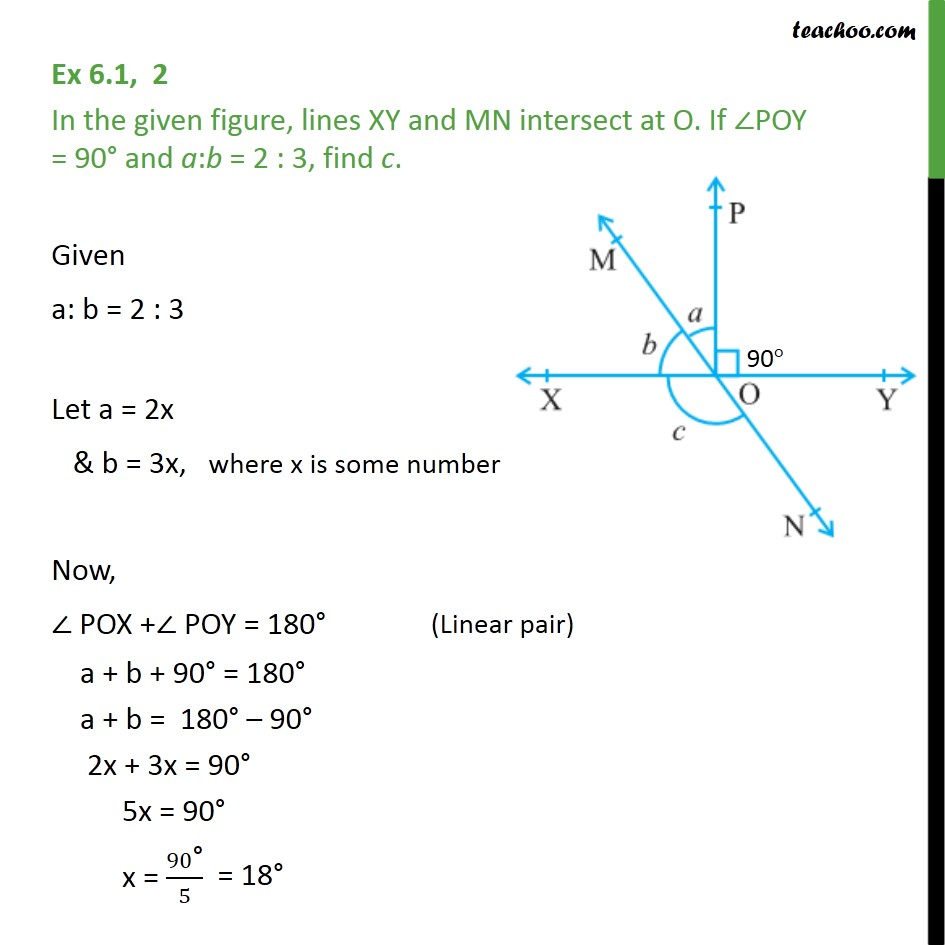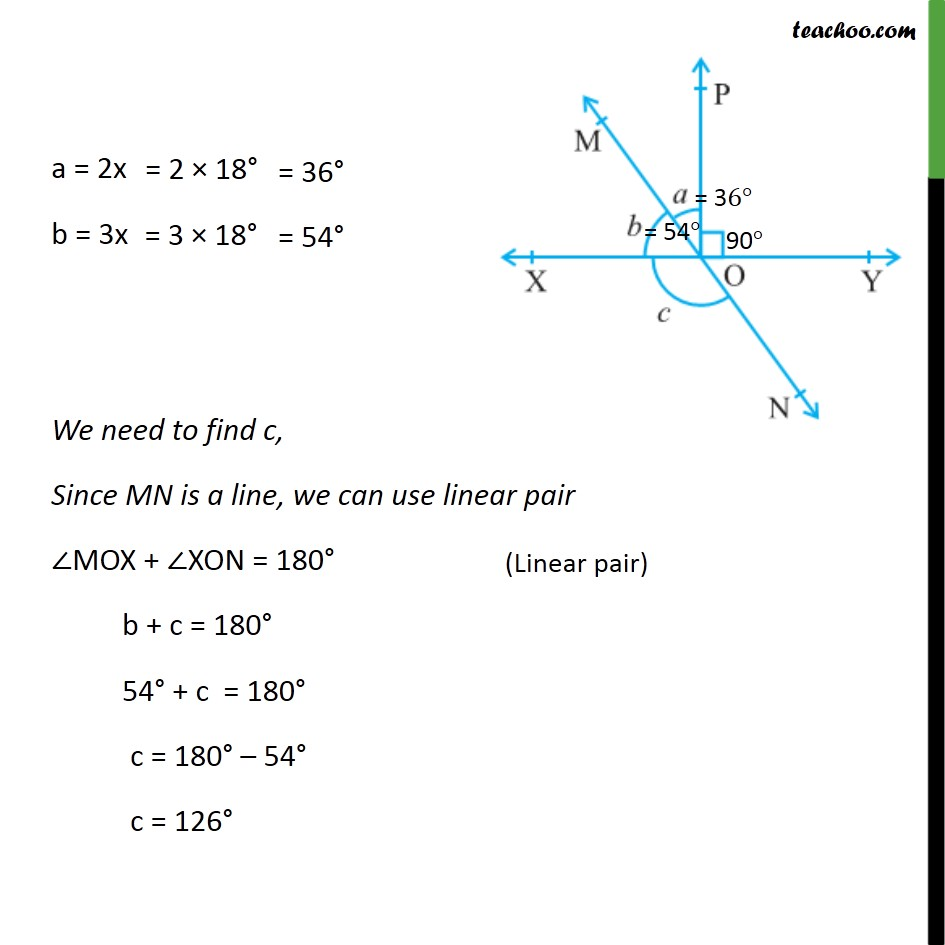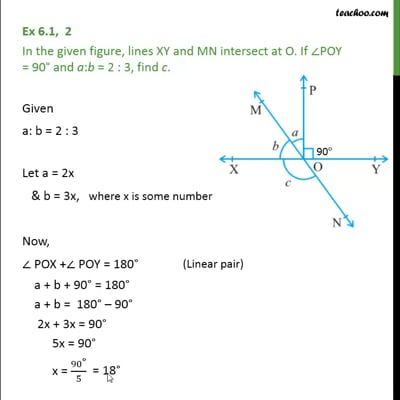Ex 6.1

Chapter 6 Class 9 Lines and Angles
Serial order wiseThis video is only available for Teachoo black users

Maths Crash Course - Live lectures + all videos + Real time Doubt solving!

### Transcript

Ex 6.1, 2 In the given figure, lines XY and MN intersect at O. If ∠POY = 90° and a:b = 2 : 3, find c. Given a: b = 2 : 3 Let a = 2x & b = 3x, where x is some number Now, ∠ POX +∠ POY = 180° a + b + 90° = 180° a + b = 180° – 90° 2x + 3x = 90° 5x = 90° x = 90"°" /5 a = 2x b = 3x We need to find c, Since MN is a line, we can use linear pair ∠MOX + ∠XON = 180° b + c = 180° 54° + c = 180° c = 180° – 54° c = 126°